SLVS815D January   2008  – October 2016

PRODUCTION DATA.

1. Features
2. Applications
3. Description
4. Revision History
5. Device Comparison Table
6. Pin Configuration and Functions
7. Specifications
8. Detailed Description
1. 8.1 Overview
2. 8.2 Functional Block Diagram
3. 8.3 Feature Description
4. 8.4 Device Functional Modes
1. 8.4.1 Soft-Start
2. 8.4.2 Power-Save Mode
9. Application and Implementation
1. 9.1 Application Information
2. 9.2 Typical Application
1. 9.2.1 Design Requirements
2. 9.2.2 Detailed Design Procedure
3. 9.2.3 Application Curves
3. 9.3 System Examples
10. 10Power Supply Recommendations
11. 11Layout
12. 12Device and Documentation Support
13. 13Mechanical, Packaging, and Orderable Information

• DDC|5

## 9 Application and Implementation

NOTE

Information in the following applications sections is not part of the TI component specification, and TI does not warrant its accuracy or completeness. TI’s customers are responsible for determining suitability of components for their purposes. Customers should validate and test their design implementation to confirm system functionality.

### 9.1 Application Information

The TPS6256x devices are high-efficiency synchronous step-down DC–DC converter featuring power-save mode or 2.25-MHz fixed frequency operation.

### 9.2 Typical Application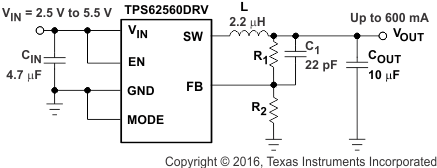Figure 6. TPS62560DRV Adjustable

#### 9.2.1 Design Requirements

The TPS6256x is a highly integrated DC/DC converter. The output voltage is set with an external voltage divider for the adjustable output voltage version. The output voltage is fixed to 1.8V for the TPS62562. For proper operation a input- and output capacitor and an inductor is required. Table 2 shows the components used for the application characteristic curves.

#### 9.2.2.1 Output Voltage Setting

For adjustable output voltage versions, the output voltage can be calculated by Equation 2 with the internal reference voltage VREF = 0.6 V typically.

Equation 2.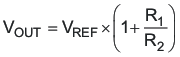To minimize the current through the feedback divider network, R2 should be 180 kΩ or 360 kΩ. The sum of R1 and R2 should not exceed ~1 MΩ, to keep the network robust against noise. An external feed-forward capacitor C1 is required for optimum load transient response. The value of C1 should be in the range between 22 pF and 33 pF.

In case of using the fixed output voltage version (TPS62562), Vout has to be connected to the feedback pin FB.

Route the FB line away from noise sources, such as the inductor or the SW line.

#### 9.2.2.2 Output Filter Design (inductor and Output Capacitor)

The TPS62560 is designed to operate with inductors in the range of 1.5 μH to 4.7 μH and with output capacitors in the range of 4.7 μF to 22 μF. The part is optimized for operation with a 2.2-μH inductor and 10-μF output capacitor.

Larger or smaller inductor values can be used to optimize the performance of the device for specific operation conditions. For stable operation, the L and C values of the output filter may not fall below 1 μH effective inductance and 3.5 μF effective capacitance.

#### 9.2.2.2.1 Inductor Selection

The inductor value has a direct effect on the ripple current. The selected inductor must be rated for its dc resistance and saturation current. The inductor ripple current (ΔIL) decreases with higher inductance and increases with higher VIN or VOUT.

The inductor selection also impacts the output voltage ripple in PFM mode. Higher inductor values lead to lower output voltage ripple and higher PFM frequency; lower inductor values lead to a higher output voltage ripple but lower PFM frequency.

Equation 3 calculates the maximum inductor current in PWM mode under static load conditions. The saturation current of the inductor should be rated higher than the maximum inductor current as calculated with Equation 4. This is recommended because during heavy load transients the inductor current rises above the calculated value.

Equation 3.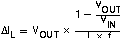Equation 4.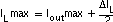where

• f = Switching frequency (2.25 MHz, typical)
• L = Inductor value
• ΔIL = Peak-to-peak inductor ripple current
• ILmax = Maximum inductor current

A more conservative approach is to select the inductor current rating just for the switch current limit ILIMF of the converter.

Accepting larger values of ripple current allows the use of lower inductance values, but results in higher output voltage ripple, greater core losses, and lower output current capability.

The total losses of the coil have a strong impact on the efficiency of the dc/dc conversion and consist of both the losses in the dc resistance (R(DC)) and the following frequency-dependent components:

• The losses in the core material (magnetic hysteresis loss, especially at high switching frequencies)
• Additional losses in the conductor from the skin effect (current displacement at high frequencies)
• Magnetic field losses of the neighboring windings (proximity effect)

### Table 1. List of Inductors

DIMENSIONS, mm INDUCTANCE, μH INDUCTOR TYPE SUPPLIER(1)
2,5 × 2 × 1 max 2 MIPS2520D2R2 FDK
2,5 × 2 × 1,2 max 2 MIPSA2520D2R2 FDK
2,5 × 2 × 1 max 2.2 KSLI-252010AG2R2 Hitachi Metals
2,5 × 2 × 1,2 max 2.2 LQM2HPN2R2MJ0L Murata
3 × 3 × 1,5 max 2.2 LPS3015 2R2 Coilcraft

#### 9.2.2.2.2 Output Capacitor Selection

The advanced fast-response voltage-mode control scheme of the TPS62560 allows the use of tiny ceramic capacitors. Ceramic capacitors with low ESR values have the lowest output voltage ripple and are recommended. The output capacitor requires either an X7R or X5R dielectric. Y5V and Z5U dielectric capacitors, aside from their wide variation in capacitance over temperature, become resistive at high frequencies.

At nominal load current, the device operates in PWM mode, and the RMS ripple current is calculated by Equation 5:

Equation 5.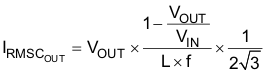At nominal load current, the device operates in PWM mode, and the overall output voltage ripple is the sum of the voltage spike caused by the output capacitor ESR plus the voltage ripple caused by charging and discharging the output capacitor shown in Equation 6:

Equation 6.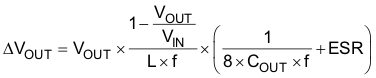At light load currents, the converter operates in power-save mode, and the output voltage ripple is dependent on the output capacitor and inductor values. Larger output capacitor and inductor values minimize the voltage ripple in PFM mode and tighten dc output accuracy in PFM mode.

#### 9.2.2.2.3 Input Capacitor Selection

An input capacitor is required for best input voltage filtering and minimizing the interference with other circuits caused by high input voltage spikes. For most applications, a 4.7-μF to 10-μF ceramic capacitor is recommended. Because a ceramic capacitor loses up to 80% of its initial capacitance at 5 V, it is recommended that 10-μF input capacitors be used for input voltages > 4.5 V. The input capacitor can be increased without any limit for better input voltage filtering. Take care when using only small ceramic input capacitors. When a ceramic capacitor is used at the input and the power is being supplied through long wires, such as from a wall adapter, a load step at the output or VIN step on the input can induce ringing at the VIN terminal. This ringing can couple to the output and be mistaken as loop instability or could even damage the part by exceeding the maximum ratings.

### Table 2. List of Capacitors(1)

CAPACITANCE TYPE SIZE SUPPLIER
4.7 μF GRM188R60J475K 0603—1,6 × 0,8 × 0,8 mm Murata
10 μF GRM188R60J106M69D 0603—1,6 × 0,8 × 0,8 mm Murata

#### 9.2.3 Application Curves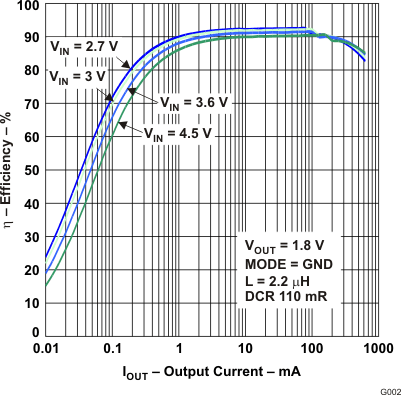Figure 7. Efficiency vs Output Current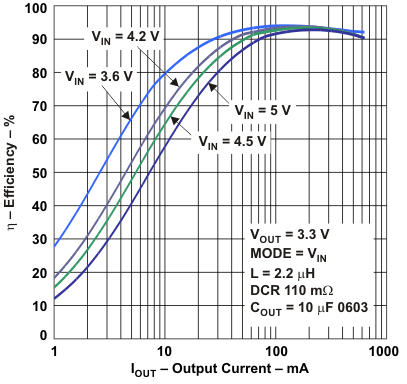Figure 9. Efficiency vs Output Current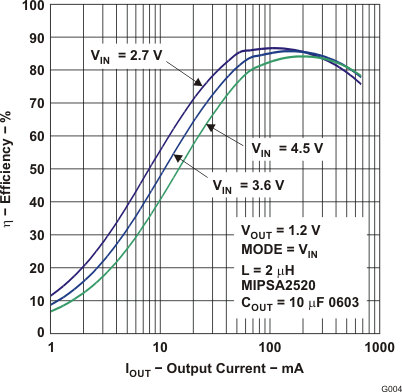Figure 11. Efficiency vs Output Current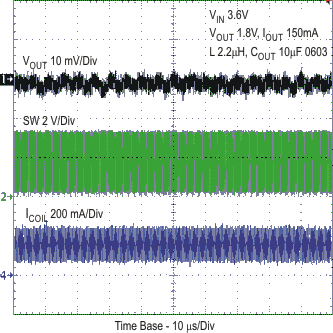Figure 13. Typical Operation - PWM Mode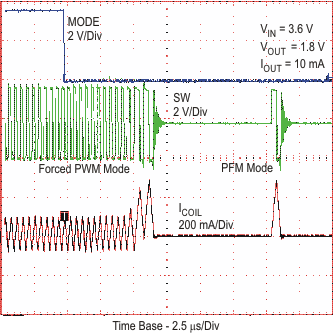Figure 15. Mode Pin Transition from PWM to PFM MODE at Light Load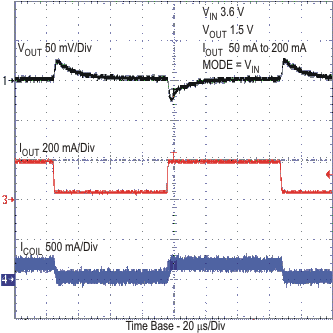Figure 17. Forced PWM Load Transient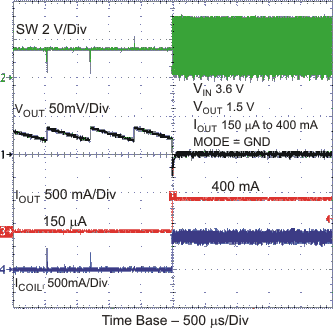Figure 19. PFM Load Transient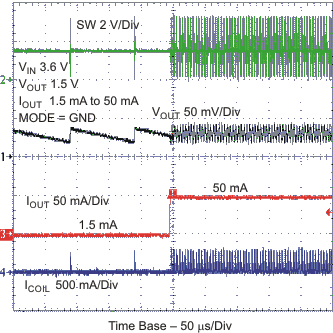Figure 21. PFM Load TransientFigure 23. PFM Load Transient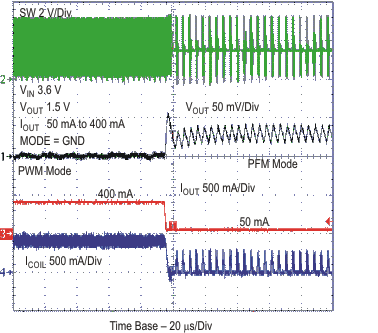Figure 25. PFM Load Transient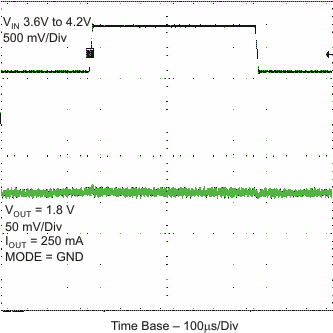Figure 27. PWM Line Transient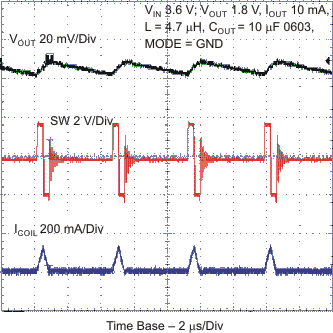Figure 29. Typical Operation - PFM Mode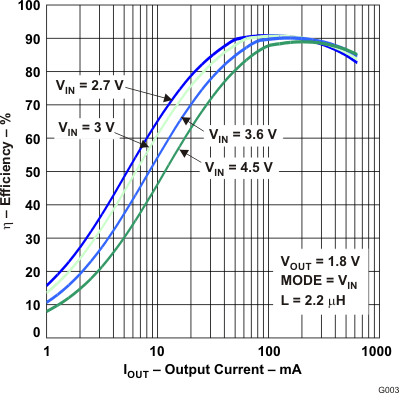Figure 8. Efficiency vs Output Current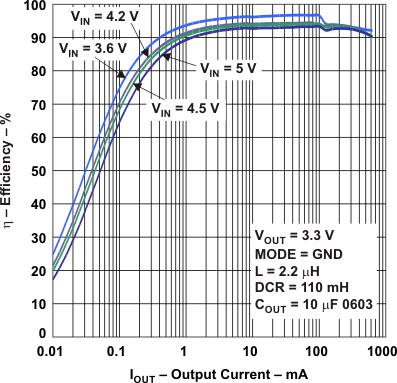Figure 10. Efficiency vs Output CurrentFigure 12. Efficiency vs Output CurrentFigure 14. Mode Pin Transition from PFM to FORCED PWM Mode at Light LoadFigure 16. Start-UP Timing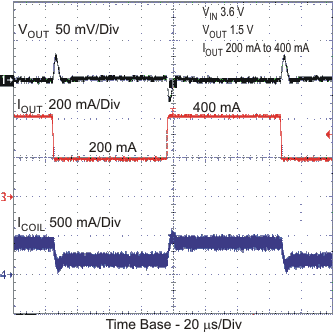Figure 18. Forced PWM Load Transient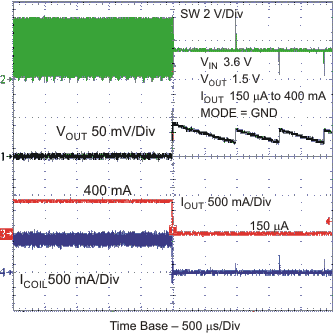Figure 20. PFM Load Transient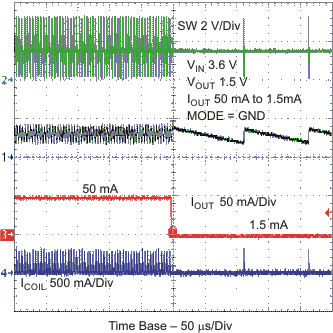Figure 22. PFM Load TransientFigure 24. PFM Load Transient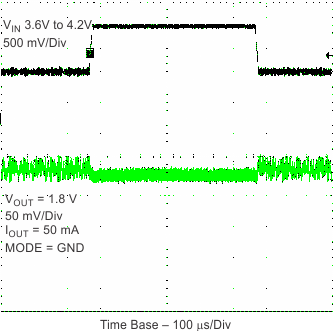Figure 26. PFM Line Transient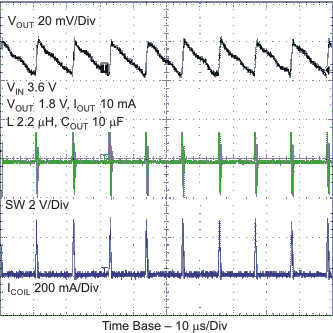Figure 28. Typical Operation - PFM Mode

### 9.3 System Examples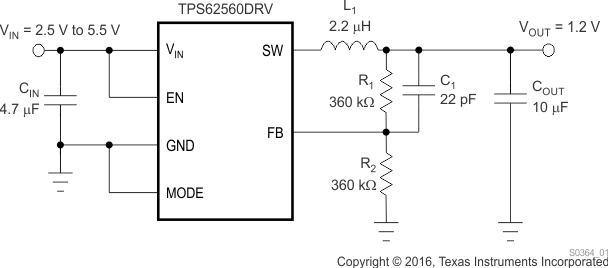Figure 30. TPS62560 Adjustable 1.2-V Output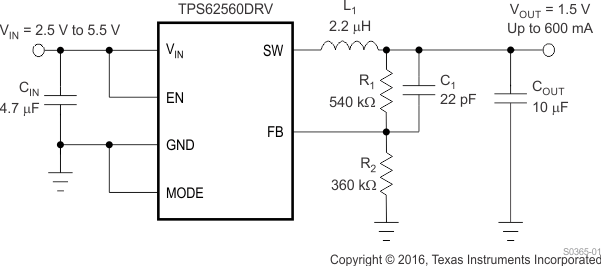Figure 31. TPS62560 Adjustable 1.5-V Output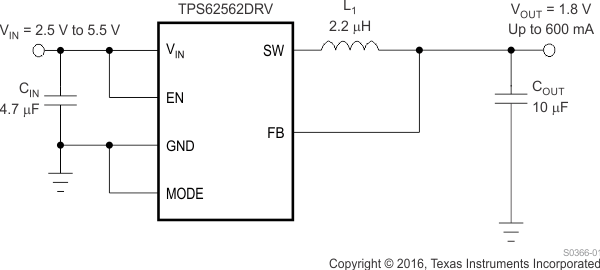Figure 32. TPS62562 Fixed 1.8-V Output